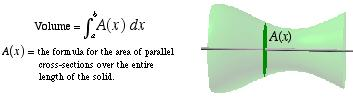index: click on a letter A B C D E F G H I J K L M N O P Q R S T U V W X Y Z A to Z index index: subject areas numbers & symbols sets, logic, proofs geometry algebra trigonometry advanced algebra & pre-calculus calculus advanced topics probability & statistics real world applications multimedia entrieswww.mathwords.com about mathwords website feedback

Volume by Parallel Cross Sections

The formula below gives the volume of a solid. A(x) is the formula for the area of parallel cross-sections over the entire length of the solid.

Note: The disk method and the washer method are both derived from this formula.Movie clips (with narration)Volume by Parallel Cross-Sections: The Concept This can be a slow download. File size is 15.2M.Volume by Parallel Cross-Sections: Example Squares on an Ellipse This can be a slow download. File size is 16.4M.

 this page updated 19-jul-17 Mathwords: Terms and Formulas from Algebra I to Calculus written, illustrated, and webmastered by Bruce Simmons Copyright © 2000 by Bruce Simmons All rights reserved